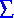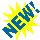### Lecture notes, etc. for:

Math 259 (Spring 1998): Introduction to Analytic Number Theory
Math 155 (Fall 1998): [Combinatorial] Designs and Groups
Math 55a (Fall 1999), Math 55b (Spring 1999-2000): Honors Advanced Calculus and Linear Algebra
Math 250 (2001-2): Higher Algebra
Math 55a (Fall 2002), Math 55b (Spring 2002-3): Honors Advanced Calculus and Linear Algebra
Math 259 (Spring 2002-3): Introduction to Analytic Number Theory (updated from the 1998-9 class)
Math 213a (Fall 2003): Complex Analysis [Functions of One Complex Variable]
Freshman Seminar 23j (Fall 2003): Chess and Mathematics
Math 250 (Fall 2004): Higher Algebra
Freshman Seminar 23j (Fall 2004): Chess and Mathematics
Math 55a (Fall 2005)
Freshman Seminar 23j (Spring [2005-]2006): Chess and Mathematics
Math 21b (Fall 2006)
Freshman Seminar 23j (Fall 2006): Chess and Mathematics
Math 259 (Spring [2006-]2007): Introduction to Analytic Number Theory (updated from the 1998-9 and 2002-3 classes)
Math 21b (Fall 2008)
Freshman Seminar 24i (Fall 2008): Mathematical Problem Solving
Freshman Seminar 24i (Fall 2009): Mathematical Problem Solving
Math 155r (Spring [2009-]2010): [Combinatorial] Designs and Groups
Math 229 (Spring [2009-]2010): Introduction to Analytic Number Theory (formerly known as Math 259, and updated from 2007 and earlier iterations)
Math 55a (Fall 2010), Math 55b (Spring 2010-11)
Freshman Seminar 24i (Fall 2010): Mathematical Problem Solving
Math 263x (Fall 2012): Computational Techniques in Number Theory and Algebraic Geometry
Math 256x (Fall 2013): The Theory of Error-Correcting Codes
Math 25b (Spring [2013-]2014): Honors Linear Algebra and Real Analysis II
Math 229 (Fall 2015): Introduction to Analytic Number Theory (formerly known as Math 259, and updated from 2009 and earlier iterations)
Math 155r (Spring [2015-]2016): [Combinatorial] Designs and Groups (updated from 2009 and earlier iterations)
Math 55a (Fall 2016)
Math 55b (Spring [2016-]2017)
Math 55a (Fall 2017)
Math 55b (Spring [2017-]2018)
Math 229 (Spring [2018-]2019): Introduction to Analytic Number Theory
Math 272 (Fall 2019): Rational Lattices and their Theta Functions
Math 229 (Spring [2019-]2020): Introduction to Analytic Number Theory
Math 229 (Spring [2020-]2021): Introduction to Analytic Number Theory

Computational number theory: Tables, links, etc.Mathematical Miscellany: Geometrical morsels, puzzles, PostScript tricks, one-page papers
here's my mathematical curriculum vitae, and a list of my math publications
Back to home page